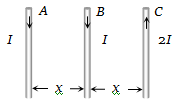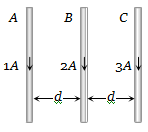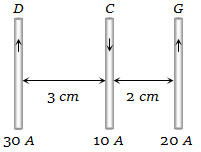Two long parallel copper wires carry currents of 5A each in opposite directions. If the wires are separated by a distance of 0.5 m, then the force between the two wires is :

(a) ${10}^{-5}N,$ attractive                (b) ${10}^{-5}N,$ repulsive

(c) $2×{10}^{-5}N,$ attractive           (d) $2×{10}^{-5}N,$ repulsive

Concept Questions :-

Force between current carrying wires
High Yielding Test Series + Question Bank - NEET 2020

Difficulty Level:

A long wire A carries a current of 10 amp. Another long wire B, Which is parallel to A and separated by 0.1m from A, carries a current of 5 amp, in the opposite direction to that in A. what is the magnitude and nature of the force experienced per unit length of B $\left({\mu }_{0}=4\mathrm{\pi }×{10}^{-7}weber/amp-\mathrm{m}\right)$

(a) Repulsive force of ${10}^{-4}N/m$

(b) Attractive force of ${10}^{-4}N/m$

(c) Repulsive force of $2\mathrm{\pi }×{10}^{-5}\mathrm{N}/\mathrm{m}$

(d) Attractive force of $2\mathrm{\pi }×{10}^{-5}\mathrm{N}/\mathrm{m}$

Concept Questions :-

Force between current carrying wires
High Yielding Test Series + Question Bank - NEET 2020

Difficulty Level:

The relation between voltage sensitivity (${\sigma }_{v}$) and current sensitivity (${\sigma }_{i}$) of a moving coil galvanometer is (Resistance of Galvanometer = G)

(a) $\frac{{\sigma }_{i}}{G}={\sigma }_{v}$                            (b) $\frac{{\sigma }_{v}}{G}={\sigma }_{i}$

(c) $\frac{G}{{\sigma }_{v}}={\sigma }_{i}$                            (d) $\frac{G}{{\sigma }_{i}}={\sigma }_{v}$

Concept Questions :-

Moving coil galvanometer
High Yielding Test Series + Question Bank - NEET 2020

Difficulty Level:

A, B, and C are parallel conductors of equal length carrying currents I, I, and 2I respectively. Distance between A and B is x. Distance between B and C is also x. ${F}_{1}$ is the force exerted by B on A and ${F}_{2}$ is the force exerted by C on A choose the correct answer:1. ${F}_{1}=2{F}_{2}$

2. ${F}_{2}=2{F}_{1}$

3. ${F}_{1}={F}_{2}$

4.

Concept Questions :-

Force between current carrying wires
High Yielding Test Series + Question Bank - NEET 2020

Difficulty Level:

Two galvanometers A and B require 3mA and 5mA respectively to produce the same deflection of 10 divisions. Then

(a) A is more sensitive than B

(b) B is more sensitive than A

(c) A and B are equally sensitive

(d) Sensitiveness of B is 5/3 times that of A

Concept Questions :-

Moving coil galvanometer
High Yielding Test Series + Question Bank - NEET 2020

Difficulty Level:

There long straight wires A, B and C are carrying current as shown figure. Then the resultant force on B is directed(a) Towards A

(b) Towards C

(c) Perpendicular to the plane of paper and outward

(d) Perpendicular to the plane of paper and inward

Concept Questions :-

Force between current carrying wires
High Yielding Test Series + Question Bank - NEET 2020

Difficulty Level:

Two long conductors, separated by a distance d carry current ${I}_{1}$ and ${I}_{2}$ in the same direction. They exert a force F on each other. Now the current in one of them is increased to two times and its direction is reversed. The distance is also increased to $3d$. The new value of the force between them is-

1. $-2F$

2.  $2F/3$

3. $-2F/3$

4. $-F/3$

Concept Questions :-

Force between current carrying wires
High Yielding Test Series + Question Bank - NEET 2020

Difficulty Level:

Current i is carried in a wire of length L. If the wire is turned into a circular coil, the maximum magnitude of torque in a given magnetic field B will be:

1. $\frac{Li{B}^{2}}{2}$                     2. $\frac{L{i}^{2}B}{2}$

3. $\frac{{L}^{2}iB}{4\mathrm{\pi }}$                     4. $\frac{L{i}^{2}B}{4\mathrm{\pi }}$

Concept Questions :-

Current carrying loop : Force and torque
High Yielding Test Series + Question Bank - NEET 2020

Difficulty Level:

Two thin, long, parallel wires, separated by a distance ‘d’ carry a current of ‘i’ A in the same direction. They will

(a) Attract each other with a force of ${\mu }_{0}{i}^{2}/\left(2{\mathrm{\pi d}}^{2}\right)$

(b) Repel each other with a force of ${\mu }_{0}{i}^{2}/\left(2{\mathrm{\pi d}}^{2}\right)$

(c) Attract each other with a force of ${\mu }_{0}{i}^{2}/\left(2\mathrm{\pi d}\right)$

(d) Repel each other with a force of ${\mu }_{0}{i}^{2}/\left(2\mathrm{\pi d}\right)$

Concept Questions :-

Force between current carrying wires
High Yielding Test Series + Question Bank - NEET 2020

Difficulty Level:

Three long, straight parallel wires carrying current, are arranged as shown in figure. The force experienced by a 25 cm length of wire C is(a) ${10}^{-3}N$

(b) $2.5×{10}^{-3}N$

(c) Zero

(d) $1.5×{10}^{-3}N$

Concept Questions :-

Force between current carrying wires